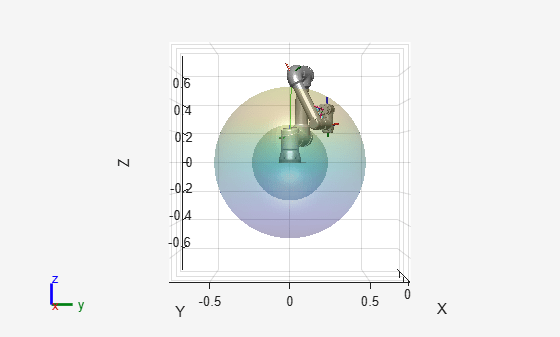# constraintDistanceBounds

Constrain body within distance bounds of reference body

Since R2022a

## Description

The `constraintDistanceBounds` object describes a constraint on the distance of one body (the end effector) relative to another body (the reference body) within the same `rigidBodyTree`. This constraint is satisfied if the distance, d, of the end effector origin relative to the reference body origin frame is within the specified bounds.

## Creation

### Syntax

``distConst = constraintDistanceBounds(endeffector)``
``distConst = constraintDistanceBounds(endeffector,Name=Value)``

### Description

example

````distConst = constraintDistanceBounds(endeffector)` returns a distance bounds constraint object, `distConst`, that represents a constraint on distance between the specified `endeffector` and the reference body specified by the `ReferenceBody` property.```
````distConst = constraintDistanceBounds(endeffector,Name=Value)` specifies properties using one or more name-value arguments.```

## Properties

expand all

Name of the end effector, specified as a string scalar or character vector. When using this constraint with a `generalizedInverseKinematics` solver, the name must match a body specified in the associated `RigidBodyTree` robot model.

Example: `"left_palm"`

Data Types: `char` | `string`

Name of the reference body frame, specified as a character vector or string scalar. The default `''` indicates that the constraint is relative to the base of the robot model. When using this constraint with a `generalizedInverseKinematics` solver, the name must match a body specified in the associated `RigidBodyTree` robot model.

Example: `"base"`

Lower and upper distance bounds imposed on the end effector from the reference body, specified as a two-element row vector of the form [minimum maximum].

Example: `[1 3]`

Weight of the constraint, specified as a numeric scalar. This weight is used with the `Weights` property of all the constraints specified in `generalizedInverseKinematics` solver to properly balance each constraint.

Example: `2`

## Examples

collapse all

Create a `constraintDistanceBounds` object and observe its effect on an inverse kinematics solution.

Load Robot and Set Up Solver

Load a Universal UR5e robot into the workspace, and create a generalized inverse kinematics solver.

```rng default; robot = loadrobot("universalUR5e",DataFormat="column"); gik = generalizedInverseKinematics("RigidBodyTree",robot);```

Set the constraint inputs `distance` for a distance bounds constraint, and `position` for the target constraint.

```gik.ConstraintInputs = {'distance','position'}; gik.SolverParameters.MaxIterations = 100;```

Create Distance Bounds Constraint

Create Distance Bounds constraint to constrain the origin of the end effector body, `tool0`, relative to the origin of the reference frame, `base`.

`constrDist = constraintDistanceBounds("tool0",ReferenceBody="base");`

Set the minimum distance between two bodies to `0.25` meters, and the maximum distance to `0.5` meters. This constraint prevents the inverse kinematics solver from solving for a configuration that violates the bounds.

```minDist = 0.25; maxDist = 0.5; constrDist.Bounds = [minDist maxDist];```

Constrain the first wrist link, `wrist_1_link`, to a target position to add some complexity.

```forearmTgt = constraintPositionTarget('wrist_1_link'); forearmTgt.TargetPosition = [0.0 0.25 0.25];```

Visualize Constraint

Run the solver through three random configurations, using the constraints, and then display the solver status. Each iteration, the solver finds a solution where the distance of the end effector is either equal to or within the specified bounds. Visualize the bounds by using the `exampleHelperVisualizeBounds` helper function to plot the distance bounds as two transparent spheres.

```for i = 1:3 figure q0 = randomConfiguration(robot); % Initial guess for solver [q,solutionInfo] = gik(q0,constrDist,forearmTgt); show(robot,q); view(90,0) hold on exampleHelperVisualizeBounds(minDist,maxDist) hold off eeDist = norm(tform2trvec(getTransform(robot,q,"tool0"))); display(["Solver Status: ",solutionInfo.Status]) display(["End Effector Distance: ",num2str(eeDist)]) end`````` 1x2 string array "Solver Status: " "success" ```
``` 1x2 string array "End Effector Distance: " "0.48425" `````` 1x2 string array "Solver Status: " "success" ```
``` 1x2 string array "End Effector Distance: " "0.29671" `````` 1x2 string array "Solver Status: " "success" ```
``` 1x2 string array "End Effector Distance: " "0.48713" ```

## Version History

Introduced in R2022a